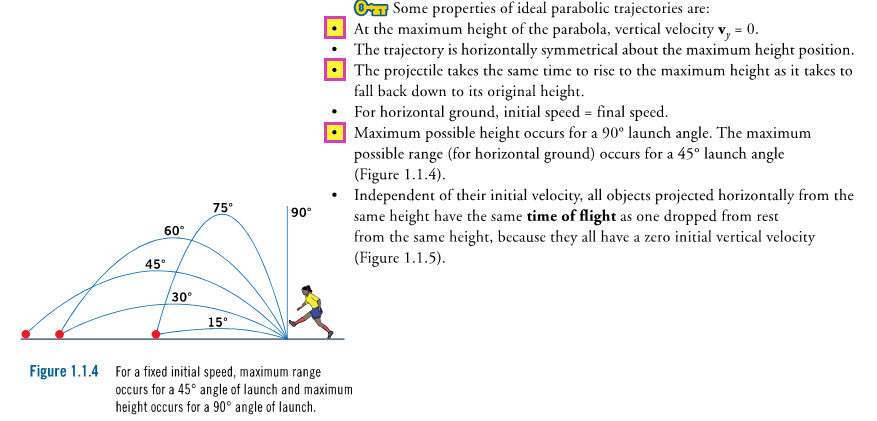# I Did the Math

Original Date: September 25, 2015

Coincidentally, I had just done the chapter on projectiles from a very awesome textbook I had recently come across.

So, I did the math.

1) Assumptions

• Zero air resistance. Ball therefore,
• travels in ideal parabolic path,
• experiences no horizontal deceleration and thus travels at a constant horizontal speed
• Catcher catches ball at same height pitcher releases it (i.e. either catcher is standing upright or pitcher is squatting)
• Distance between pitcher and catcher is 18.4 meters (Wikipedia)
• Also these three facts from the textbook,2) Work with what we have,

Vertical

Time ball takes to reach midpoint,

Horizontal

Time ball takes to reach catcher,

3) Combine the two worlds,

4) Americanize the units,